# Radio Antenna Erection and Standing Wave Ratio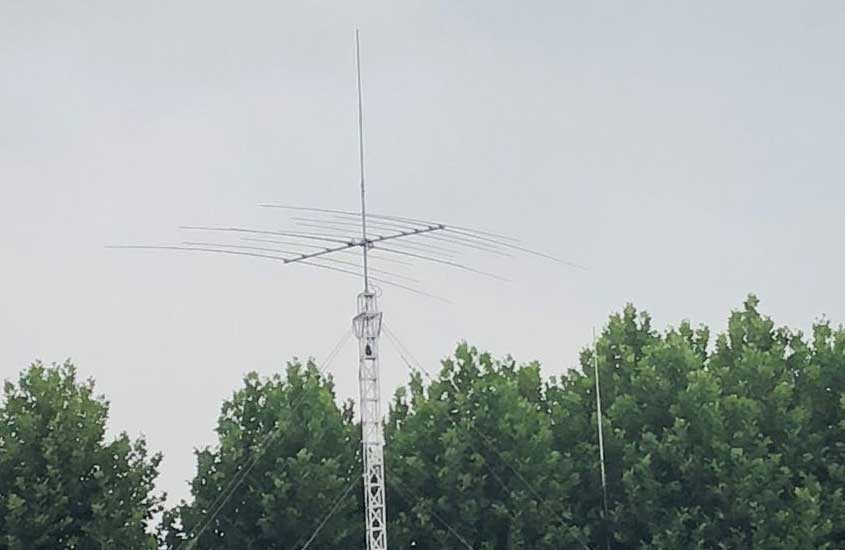Voltage standing wave ratio (VSWR) is the most commonly used parameter in RF technology to measure the good match between components. When amateur radio enthusiasts contact, of course, first think about measuring whether the VSWR of the antenna system is close to 1:1. If it is close to 1:1, it is good. I often hear this question: But what if I can't reach 1? If the standing wave ratio is as small as a few, is the antenna qualified?

## VSWR and Nominal Impedance

The condition that the transmitter and the antenna are matched is that the resistance components of the impedance of the two are the same, and the inductive reactance portions cancel each other. If the impedance of the transmitter is different, the impedance of the antenna is required to be different. In the era of the electron tube, on the one hand, the output impedance of the tube is high. On the other hand, the low-impedance coaxial cable has not been popularized. The popular one is a parallel feeder with a characteristic impedance of several hundred ohms. Therefore, the output impedance of the transmitter is several hundred ohms. The nominal impedance of antennas for modern commodity solid-state radios is 50 ohms, so the commercial VSWR meter is also scaled to 50 ohms.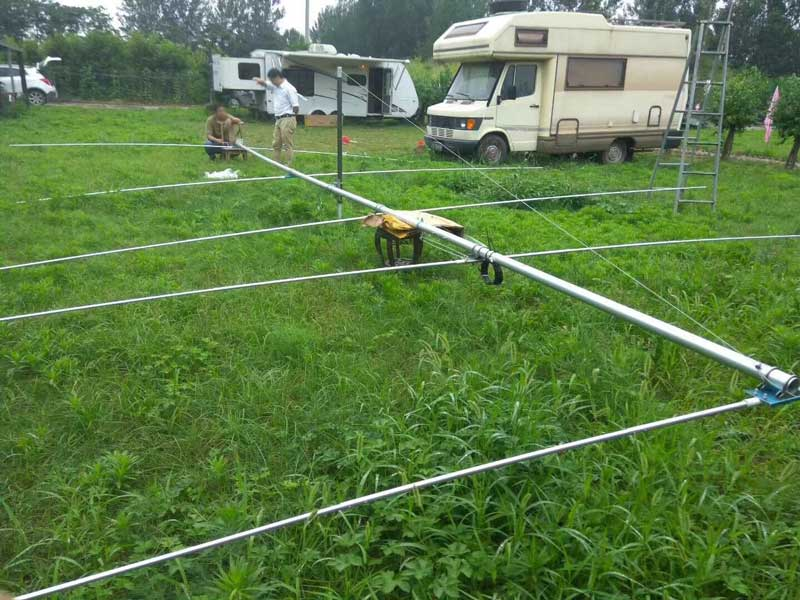## 1. VSWR is not equal to 1

When VSWR is not 1, it does not make sense to compare the value of VSWR.

The antenna VSWR=1 indicates that the antenna system and the transmitter satisfy the matching condition, and the energy of the transmitter can be most efficiently transmitted to the antenna, and the matching situation is only one. And if VSWR is not equal to 1, for example, equal to 4, then there are many possibilities: antenna inductive detuning, antenna capacitive detuning, antenna resonance but feed point is wrong, and so on. On the impedance map, each VSWR value is a garden with an infinite number of points. That is to say, when the VSWR values are the same, there are many possibilities for the state of the antenna system. Therefore, it is not too strict to use the VSWR values for a simple comparison between the two antennas.

VSWR values other than 1 are not so accurately determined (unless there are special needs), so most VSWR tables are not as carefully calibrated as voltmeters and ohmmeters, and even few VSWRs give their error level data. Due to the phase frequency characteristics of the RF coupling components in the table and the nonlinearity of the diode, the error of most VSWR meters at different frequencies and different powers is not uniform.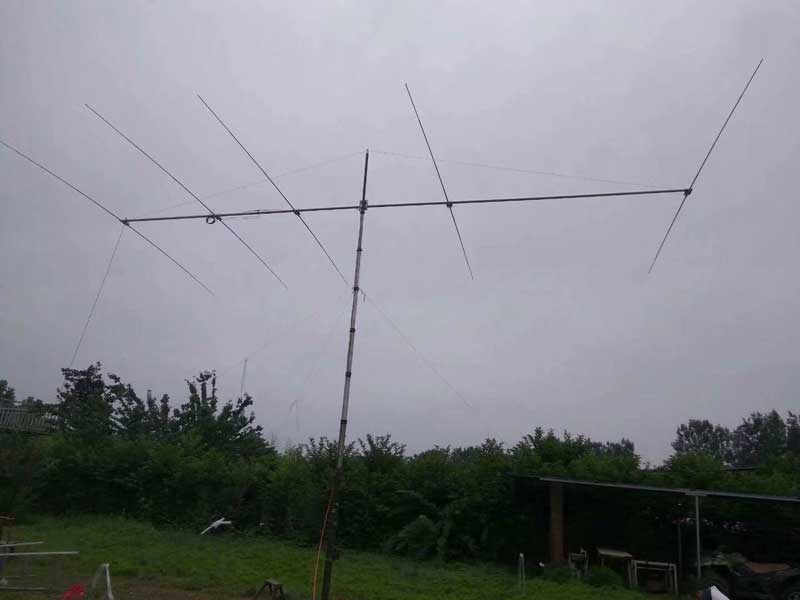## 2. VSWR is equal to 1

VSWR = 1 does not equal to a good antenna.

Some foreign magazine articles often give a VSWR curve when introducing an antenna. Sometimes it creates an illusion that as long as VSWR=1, it will always be a good antenna. In fact, VSWR=1 only indicates that the transmitter's energy can be efficiently transmitted to the antenna system. But whether this energy can be effectively radiated into space is another problem. A dipole antenna made according to the theoretical length and a shortened antenna with a length of only 1/20, as long as appropriate measures are taken, they may all achieve VSWR=1, but the launching effect is definitely quite different and cannot be said in the same breath. As an extreme example, a 50ohm resistor whose VSWR is ideally equal to 1, but its emission efficiency is zero.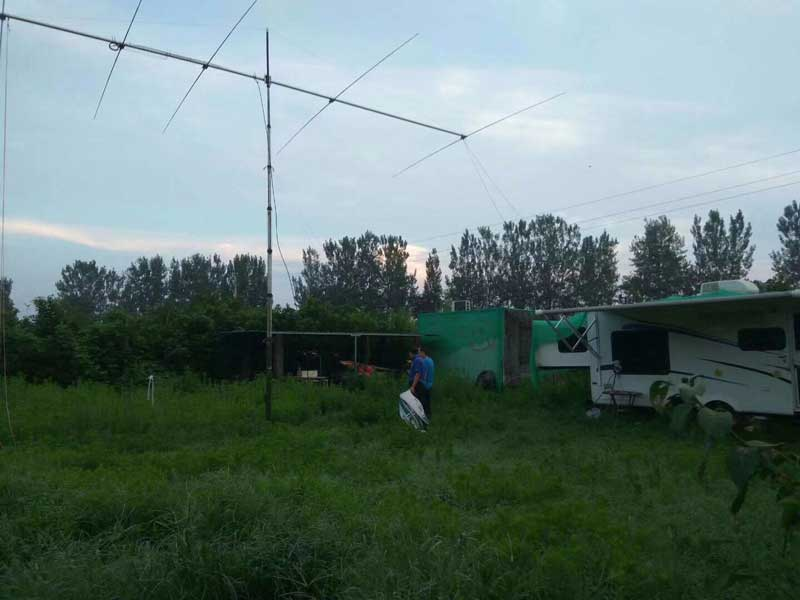### The most important factor affecting the antenna effect: Resonance

The matching condition of the antenna system and the transmitter with an output impedance of 50 ohms is that the impedance of the antenna system is 50 ohms. To meet this condition, two things need to be done: First, the antenna circuit resonates with the operating frequency (otherwise the antenna impedance is not a pure resistance); second, select the appropriate feed point.

Let us illustrate with the strings of stringed instruments. Whether it is a violin or a guzheng, each of its strings has its own natural frequency for a specific length and tension. When the string vibrates at the natural frequency, both ends are fixed and cannot move, but the tension in the vibration direction is the largest. The middle swing is the largest, but the vibration tension is the slackest. This is equivalent to a free-resonant antenna with a total length of 1/2 wavelength. There is no current (current valley) at both ends and the voltage amplitude is the largest (voltage antinode), the intermediate current is the largest (current antinode) and the voltage at the two adjacent points is the smallest. (Voltage trough).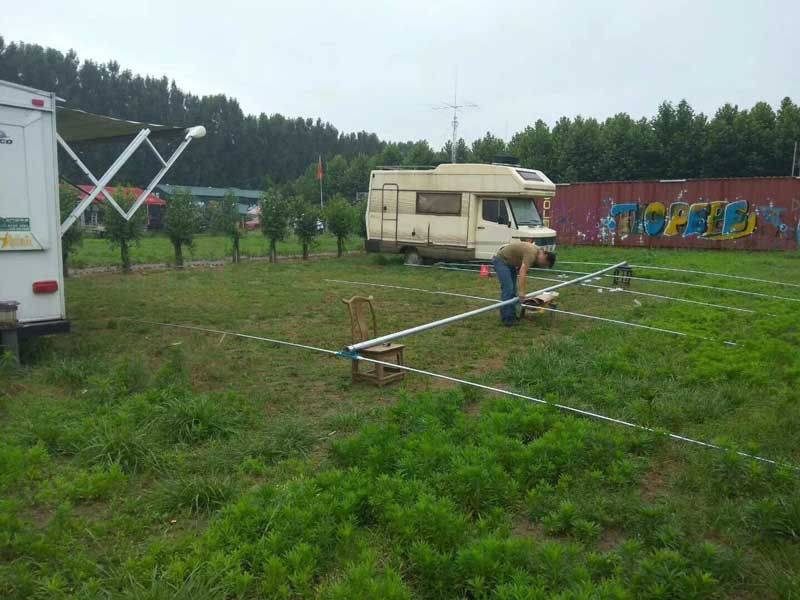We want to make the string produce the strongest sound. First, the desired sound can only be the natural frequency of the string. Second, the ratio of the tension of the driving point to the swing amplitude should be appropriate, that is, the impedance of the driving source to the driving point on the string. match. The specific performance is that the bow of the string or the finger of the plucking should be selected at the appropriate position of the string. In practice, it is not difficult to find that the wrong position of the bow or the plucking string will affect the vocal intensity of the string, but the slightest improperness will not affect too much, but it is very difficult to make a sound different from the natural frequency of the string. At this time, the vibration state of each point on the string is very complicated and chaotic. Even if it vibrates, the push of each point to the air is not united, and the sounding efficiency is very low.

The same is true for the antenna. The electromagnetic field emitted by the antenna must be the strongest. First, the transmission frequency must be the same as the natural frequency of the antenna. Second, the driving point should be selected at the appropriate position of the antenna. If the driving point is not appropriate and the antenna resonates with the signal frequency, the effect will be slightly affected, but if the antenna does not resonate with the signal frequency, the transmission efficiency will be greatly reduced.

Therefore, in the two points that antenna matching needs to be done, resonance is the most critical factor.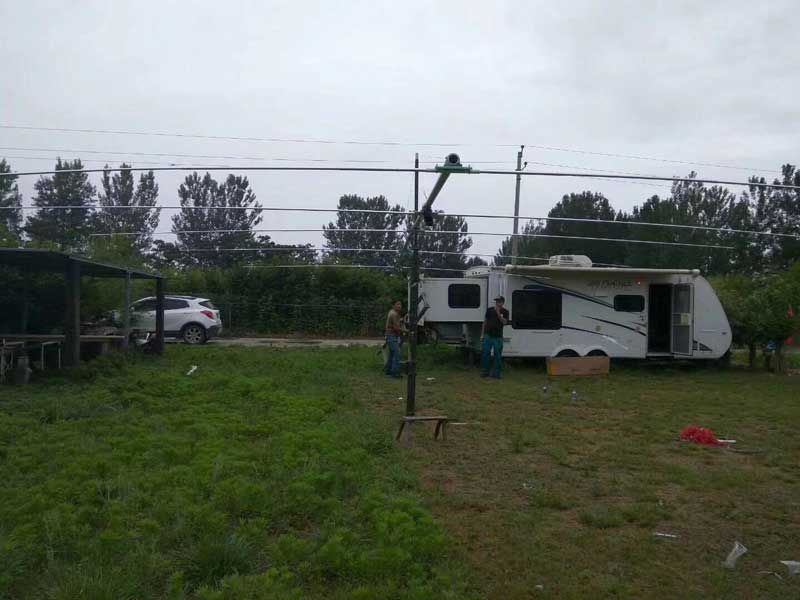### Standing wave ratio of antenna and standing wave ratio of the antenna system

The VSWR of the antenna needs to be measured at the feed end of the antenna. But the antenna feed point is often high in the air, we can only measure the VSWR at the lower end of the antenna cable so that the VSWR of the entire antenna system including the cable is measured. When the impedance of the antenna itself is indeed 50 ohms pure resistance and the characteristic impedance of the cable is indeed 50 ohms, the measured result is correct.

When the antenna impedance is not 50 ohms and the cable is 50 ohms, the measured VSWR value will be seriously affected by the length of the antenna. Only when the electrical length of the cable is exactly one multiple of the wavelength, and the cable loss can be ignored, the lower end of the cable The impedance presented is exactly the same as the impedance of the antenna. But even if the cable length is a multiple of the wavelength, but the cable is lossy, for example, the cable is thinner, the electrical length of the cable reaches dozens of times the wavelength, then the VSWR measured at the lower end of the cable will be lower than the actual VSWR of the antenna.

Therefore, when measuring VSWR, especially in the UHF band, do not ignore the impact of the cable.